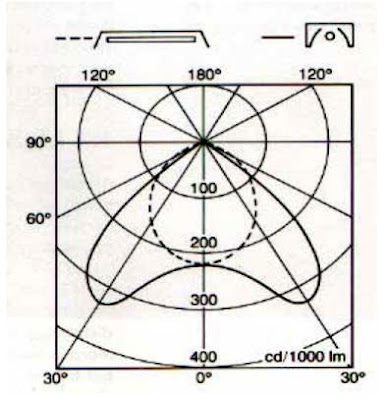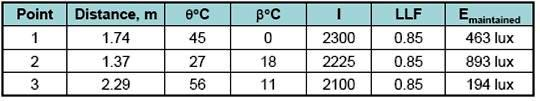Point by Point Method for Lighting Design

I indicted before in our course   that we can design interior lighting by using any method from the following three ones:

1. The Zonal Cavity (Lumen) method,
2. Point by point method,
3. Watt per square feet method.

Today I will explain the second method for lighting design which is “ point by point method” as follows .

You can review the following previous articles for more information and good following:

Second: Point by Point Method

The first method for lighting design which was Zonal cavity (Lumen) method is used to calculate average illuminance for places having uniform lighting distrbution, but this method , for example, can’t answer the following questions:

1. What is the illuminance on a wall display from a spotlight aimed at the display?
2. How much light is striking a point on the façade of a building or in a parking lot from a floodlight?

The only way for getting answers for above questions is by applying point to point method for lighting design.

Three Factors must be considered for applying the Point by point method:

1. Luminous intensity,
2. Distance,
3. Orientation of the surface.

1- Luminous Intensity (I)

The luminous intensity (candelas) is the strength (intensity) of the light produced in a specific direction.

Or

It is Luminous Flux in a certain direction, radiated per unit of solid angle.

It’s unit is candela.The luminous intensity of any light source is compiled graphically into diagrams known as candela or candlepower distribution curves. Both polar and Cartesian graphs are used within the lighting industry for this purpose. This information is also available in numeric tabular form.

Candlepower Distribution Curve

A curve, generally polar, represents the variation of luminous intensity of a lamp or luminaire in a plane through the light center.

The luminous intensity (I) is determined using the photometric data for the specific luminaire used and the angular relationship between the luminaire aiming direction and the direction from the luminaire to the calculation point.

There are three types of Candlepower Distribution Curve as follows:

A- Rotational symmetrical

Light distribution same in all planes. Usually Circular or ‘Bowl shaped’ luminaireRotational symmetrical

B- Planar symmetrical

Luminaire distribution is confined to two vertical planes separately. Typical distribution for Fluorescent Lamp luminaires and Road LightingPlanar symmetrical

C- Asymmetrical

Asymmetry present in one of the Planes of measurement.Asymmetrical

2- Distance

Distance between a surface and the source affects the illuminance (luminous flux per unit of area) striking that surface

Surface of a given area that is closer to the source captures a larger portion of the flux in the cone than a surface of the same given area that is further away

Considering the luminous intensity as the luminous flux (lumens) leaving a source in a cone traveling in a specific direction, as the area increases the iluminance decreases while the luminous flux remains the same.

Inverse Square Law

It states that the cross-sectional area of the cone increases with the square of the distance from the source.

Therefore, the illuminance on this surface varies inversely with the square of the distance from the source.Inverse Square Law states that

E = I/ d2
Where:

E = Illuminance on the surface

I = Luminous intensity of the source in the direction of the surface

d = Distance from the source to the surface

3- Orientation of the Surface

Surface orientation is included in the Inverse Square Law by adding a cos θ term:

E = I/ d2 cos θ

Where:
θ is the angle between the light ray coming from the source to the point, and a line that is perpendicular (normal) to the plane or surface on which the illuminance is being measured or calculated (see fig.).

Example #1:

This example will consider the illuminance at a single point on a horizontal surface from a single luminaire straight down, given that:

• D = 2.13 m
• θ = 15°
• LLF = 0.85
• I = 2200 candelas

Calculate the illuminance level on this point.

Using the equation;

E = I/ d2 x cos θ x LLFTOTAL

E = 2200 cd x cos 15° x 0.85 / 2.13 m2

E = 398 lux (maintained)

This tells us that 398 lux will strike the point in question directly from the luminaire and no reflected light is calculated. The answer is a maintained illuminance level since a light loss factor of 0.85 was included to account for the loss of light over time due to reduced lumen output of the lamp and dirt on the luminaire surfaces.

point by point method calculations for different cases:

Based on the orientation of the surface, we have (5) cases in application of point to point method for lighting design as follows:

1. Illuminance directly below the luminaire on a horizontal surface,
2. Illuminance on horizontal surface but at angle to luminaire,
3. Illuminance on vertical surface at angle to luminaire,
4. Illuminanceon a tilted or sloped surface,
5. Illuminanceon for multiple point source calculations.

1- Illuminance directly below the luminaire on a horizontal surface

2- Illuminance on horizontal surface but at angle to luminaire

3- Illuminance on vertical surface at angle to luminaire

4- Illuminanceon a tilted or sloped surface

5- Illuminanceon for multiple point source calculations (
Abney’s Law )Example 2:

This example will consider the illuminance at a single point on a horizontal surface from two luminaires aimed straight down. An assumed LLF of 0.85 will be used and Luminaire #1 is the same in Example#1 and given that:

• D1 = 2.13m,  θ1 = 15°
• D2 = 2.29m,  θ2 = 25°
• β1 = 15°,  I1 = 2200 cd
• β2 = 25°,  I2 = 2000 cd
Calculate the illuminance level on this point.

E1 = 398 lux (from previous calculation in example#1)

E2 = 291 lux (same calculation method as E1)

Hence, Illumination from more than one source is added arithmetically
So, E total = E1 + E2 = 689 lux

Example 3:

This example will consider the illuminance at multiple points on a vertical surface from a luminaire aimed at the surface. An assumed LLF of 0.85 will be used. The data for three points 1, 2& 3 are listed in below table:Calculate the illuminance level on the three points.

The luminaire is now aimed at the vertical surface so β is no longer measured from straight down, and β and θ are no longer equal.

Illuminance is calculated using the same equation as the prior examples , the results are listed in below tableNotes for example#3:

• In Table 1, illuminance at point 2 is greater than at point 1 and illuminance at point 3 is the least. This is because the distance at point 2 is less than point 1 and the angle theta (θ ) at point 2 is less than at point 1, despite the fact that the intensity in that direction is less.
• Similar reasoning can be used with regard to point 3.
• These two factors cause the illuminance at point 2 to be greater than the illuminance at point 3.

Limits for using point by point method:

• Maximum physical dimension of the surface under design is not larger than 1/5th the mounting height above the evaluation point.
• Does not apply to a surface of infinite length.

In the next Article, I will explain the third method for lighting design “Watt per square feet method”. Please, keep following.